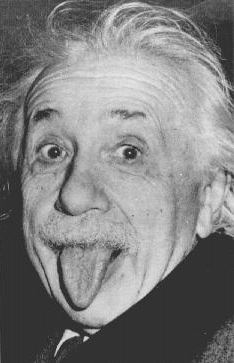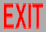# Special relativity made stupid ☺️

Many laymen seem to be antagonistic towards Einstein's Theory of Special Relativity, either because they find it too complicated, or because they think it contradicts common knowledge. In fact, special relativity is barely more complicated than Euclidean geometry, as we describe below.

## Measuring distances in Euclidean geometry

The Pythagorean theorem for three-dimensional space says that if you have a box of width "x", length "y", and height "z", the distance "s" between opposite corners is given by

s2 = x2 + y2 + z2

In general, if you want to measure the distance between two points, you can just line up a ruler directly between the two points and measure it. However, for practical reasons, it might be easier to imagine a box, which is really just saying you measure distances along three perpendicular axes: For example, if one point is above the ground and the other on it, you can first measure the altitude of the first point directly above a point on the ground, and then measure distance on the ground from there to the second point. Then the Pythagorean theorem tells you how to calculate the direct distance. In principle, if you just have two points, and nothing else to worry about, then it doesn't matter how you choose the axes (how you orient the "box", which will have different dimensions for different orientations): All that matters is the resulting distance "s", not the separate "x", "y", and "z" ("Cartesian coordinates"): You can rotate your axes any way you want. The same method applies to measuring the distance along a curve: You divide the curve into a bunch of small, almost-straight pieces, and add up the lengths.

## Measuring distances in special relativitySpace and time describe a four-dimensional "spacetime". For example, if you ignore two of the dimensions of space, you can plot the motion of an object on a graph, with its position along one axis and the time along another. You then think of this graph as a picture in two-dimensional spacetime. The object now has an extra dimension to it: For example, if the original object was a point in space, it is now a curve in spacetime, each point along the curve representing that object at a different time.

According to special relativity, the "distance" along this curve is measured differently; it is

-s2 = -t2 + x2 + y2 + z2

This distance is called the "proper time": It is the time measured by a clock moving along the straight line between the two points. (Clocks are used for measurements in special relativity.) Just as distance in Euclidean space doesn't depend on how you choose your axes, proper time doesn't depend on how you choose all four axes: You can rotate the three space axes among themselves, but you can also rotate between time and space; this is called a "Lorentz transformation". But Lorentz transformations are limited in that you can't "rotate" far enough to switch the signs in the expression for "s2" above. It is clear from this picture that the time measured by a clock depends on what path it took between the two points.

"Simultaneity" is often confusing to people first learning special relativity, since it no longer exists. But that should be clear from the above picture: If you rotate a picture on your desk, then points that were level will no longer be so. Likewise, if you rotate the spacetime picture discussed above, points that were at the same time will not be.A famous "paradox" is trying to park a relativistic car in a garage: From the point of view of the car, the garage has "Lorentz contracted", and the car will no longer fit. But from the point of view of the garage, the car is now shorter, and so will fit even better. The resolution of the paradox is that if the front end of the car stops simultaneously to the back end from one "reference frame", that will not be true in the other. If both ends do not stop at the same time, the car changes length. (This has often been observed nonrelativistically, for cars stopped by trees or other cars.)